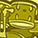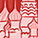Announcements

#### Curious on how you can apply Gate Driver ICs to Smart Home Appliances? Register for the ASEAN Smart Home Appliance Webinar here to find out how.

Tip / Sign in to post questions, reply, level up, and achieve exciting badges. Know more

# Gate Driver ICs Forum DiscussionsLevel 1
Level 1

# 1EBN1001AE-Booster IC power dissipation calculation

Hello ,

We would like to calculate the power dissipation of the Booster IC 1EBN1001AE.After going through the datasheet we didn't find any detailed procedure to calculate power dissipation of the IC.Kindly
help us to calculate the power dissipation of the IC.
1 SolutionModeratorModerator

# Re: 1EBN1001AE-Booster IC power dissipation calculation

Hi,

Since, data sheet doesn't contain the process to calculate the power loss, I will recommend you the general practice to calculate power loss. This procedure is a mixture of experimental result and data sheet values.

1) Test the IC at rated conditions/maximum operating condition at a particular required ambient temperature and measure the case temperature. By this method you will get the temperature rise or temperature difference.

2) Refer to data sheet of the heat sink paste and note the Rth (equivalent thermal resistance ) value and the Rth from IC data sheet (if any from case to local body ). In case you are measuring temperature at the case (no local body present) then you can take only heat sink paste Rth.

3) After noting down the values, apply basic formula to calculate the approximate power loss.

Y rise = Rth (equivalent) × Ploss

BR,

AZIZ HASSAN

2 RepliesModeratorModerator

# Re: 1EBN1001AE-Booster IC power dissipation calculation

Hi,

Kindly contact your regional Infineon team for support on the automotive grade gate driver - 1EBN1001AE.

Thanks and regards,

SrivatsaModeratorModerator

# Re: 1EBN1001AE-Booster IC power dissipation calculation

Hi,

Since, data sheet doesn't contain the process to calculate the power loss, I will recommend you the general practice to calculate power loss. This procedure is a mixture of experimental result and data sheet values.

1) Test the IC at rated conditions/maximum operating condition at a particular required ambient temperature and measure the case temperature. By this method you will get the temperature rise or temperature difference.

2) Refer to data sheet of the heat sink paste and note the Rth (equivalent thermal resistance ) value and the Rth from IC data sheet (if any from case to local body ). In case you are measuring temperature at the case (no local body present) then you can take only heat sink paste Rth.

3) After noting down the values, apply basic formula to calculate the approximate power loss.

Y rise = Rth (equivalent) × Ploss

BR,

AZIZ HASSAN